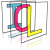Image Component Library (ICL)
icl::math::DynMatrix< T >::DynMatrixColumn Class Reference

Internally used Utility structure referencing a matrix column shallowly. More...

`#include <DynMatrix.h>`

## Public Member Functions

DynMatrixColumn (const DynMatrix< T > *matrix, unsigned int column)
create from source matrix and column index More...

DynMatrixColumn (const DynMatrix< T > &matrix)
Create from source matrix (only works if matrix has only single column = column-vector) More...

DynMatrixColumn (const DynMatrixColumn &c)
Shallow copy from another matrix column reference. More...

col_iterator begin ()
returns column begin More...

col_iterator end ()
returns column end More...

const col_iterator begin () const
returns column begin (const) More...

const col_iterator end () const
returns column end (const) More...

unsigned int dim () const
returns column length (matrix->rows()) More...

DynMatrixColumnoperator= (const DynMatrixColumn &c)
assignment by another column More...

DynMatrixColumnoperator= (const DynMatrix &src)
assigne dyn matrix to matrix columns More...

DynMatrixColumnoperator+= (const DynMatrixColumn &c)
operator += for other columns More...

DynMatrixColumnoperator-= (const DynMatrixColumn &c)
operator += for other columns More...

DynMatrixColumnoperator+= (const DynMatrix &m)
operator += for DynMatrices More...

DynMatrixColumnoperator-= (const DynMatrix &m)
operator -= for DynMatrices More...

DynMatrixColumnoperator *= (const T &val)
operator *= for scalars More...

DynMatrixColumnoperator/= (const T &val)
operator /= for scalars More...

## Public Attributes

DynMatrix< T > * matrix
Matrix reference. More...

unsigned int column
referenced column in matrix More...

## Detailed Description

### template<class T> class icl::math::DynMatrix< T >::DynMatrixColumn

Internally used Utility structure referencing a matrix column shallowly.

## ◆ DynMatrixColumn() [1/3]

template<class T>
 icl::math::DynMatrix< T >::DynMatrixColumn::DynMatrixColumn ( const DynMatrix< T > * matrix, unsigned int column )
inline

create from source matrix and column index

## ◆ DynMatrixColumn() [2/3]

template<class T>
 icl::math::DynMatrix< T >::DynMatrixColumn::DynMatrixColumn ( const DynMatrix< T > & matrix )
inline

Create from source matrix (only works if matrix has only single column = column-vector)

## ◆ DynMatrixColumn() [3/3]

template<class T>
 icl::math::DynMatrix< T >::DynMatrixColumn::DynMatrixColumn ( const DynMatrixColumn & c )
inline

Shallow copy from another matrix column reference.

## ◆ begin() [1/2]

template<class T>
 col_iterator icl::math::DynMatrix< T >::DynMatrixColumn::begin ( )
inline

returns column begin

## ◆ begin() [2/2]

template<class T>
 const col_iterator icl::math::DynMatrix< T >::DynMatrixColumn::begin ( ) const
inline

returns column begin (const)

## ◆ dim()

template<class T>
 unsigned int icl::math::DynMatrix< T >::DynMatrixColumn::dim ( ) const
inline

returns column length (matrix->rows())

## ◆ end() [1/2]

template<class T>
 col_iterator icl::math::DynMatrix< T >::DynMatrixColumn::end ( )
inline

returns column end

## ◆ end() [2/2]

template<class T>
 const col_iterator icl::math::DynMatrix< T >::DynMatrixColumn::end ( ) const
inline

returns column end (const)

## ◆ operator *=()

template<class T>
 DynMatrixColumn& icl::math::DynMatrix< T >::DynMatrixColumn::operator *= ( const T & val )
inline

operator *= for scalars

## ◆ operator+=() [1/2]

template<class T>
 DynMatrixColumn& icl::math::DynMatrix< T >::DynMatrixColumn::operator+= ( const DynMatrixColumn & c )
inline

operator += for other columns

## ◆ operator+=() [2/2]

template<class T>
 DynMatrixColumn& icl::math::DynMatrix< T >::DynMatrixColumn::operator+= ( const DynMatrix & m )
inline

operator += for DynMatrices

## ◆ operator-=() [1/2]

template<class T>
 DynMatrixColumn& icl::math::DynMatrix< T >::DynMatrixColumn::operator-= ( const DynMatrixColumn & c )
inline

operator += for other columns

## ◆ operator-=() [2/2]

template<class T>
 DynMatrixColumn& icl::math::DynMatrix< T >::DynMatrixColumn::operator-= ( const DynMatrix & m )
inline

operator -= for DynMatrices

## ◆ operator/=()

template<class T>
 DynMatrixColumn& icl::math::DynMatrix< T >::DynMatrixColumn::operator/= ( const T & val )
inline

operator /= for scalars

## ◆ operator=() [1/2]

template<class T>
 DynMatrixColumn& icl::math::DynMatrix< T >::DynMatrixColumn::operator= ( const DynMatrixColumn & c )
inline

assignment by another column

## ◆ operator=() [2/2]

template<class T>
 DynMatrixColumn& icl::math::DynMatrix< T >::DynMatrixColumn::operator= ( const DynMatrix & src )
inline

assigne dyn matrix to matrix columns

## ◆ column

template<class T>
 unsigned int icl::math::DynMatrix< T >::DynMatrixColumn::column

referenced column in matrix

## ◆ matrix

template<class T>
 DynMatrix* icl::math::DynMatrix< T >::DynMatrixColumn::matrix

Matrix reference.

The documentation for this class was generated from the following file:
• /Users/alneuman/vm/icl/ICLMath/src/ICLMath/DynMatrix.h